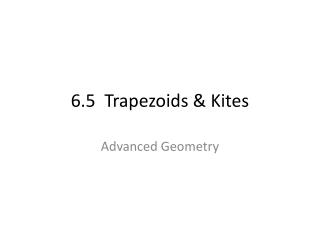# 6.5 Trapezoids & Kites - PowerPoint PPT PresentationDownload Presentation6.5 Trapezoids & Kites

6.5 Trapezoids & KitesDownload Presentation## 6.5 Trapezoids & Kites

- - - - - - - - - - - - - - - - - - - - - - - - - - - E N D - - - - - - - - - - - - - - - - - - - - - - - - - - -
##### Presentation Transcript

1. 6.5 Trapezoids & Kites Advanced Geometry

2. TRAPEZOID A trapezoid is a quadrilateral with exactly one pair of parallel sides.

3. Parts of a Trapezoid • The parallel sides are the bases • A trapezoid has two pairs of base angles • The nonparallel sides are the legs of the trapezoid

4. ISOSCELES TRAPEZOID If the legs of a trapezoid are congruent, then the trapezoid is an isosceles trapezoid.

5. THEOREM 6.14 If a trapezoid is isosceles, then each pair of base angles is congruent.

6. THEOREM 6.15 If a trapezoid has a pair of congruent base angles, then it is an isosceles trapezoid.

7. THEOREM 6.16 A trapezoid is isosceles if and only if its diagonals are congruent.

8. Example CDEF is an isosceles trapezoid with CE = 10 and measure of angle E = 95 degrees. Find DF, measure of angle C, D, and F.

9. How? If we were given the graph of a quadrilateral, how would we prove that it is a trapezoid? How would we prove that it is an isosceles trapezoid?

10. Example The vertices of WXYZ are W(-1,2), X(3,0), Y(4,-3), and Z(-4,1). Show that WXYZ is an isosceles trapezoid.

11. MIDSEGMENT OF A TRAPEZOID The midsegment of a trapezoid is the segment that connects the midpoints of its legs.

12. MIDSEGMENT THEOREM FOR TRAPEZOIDS The midsegment of a trapezoid is parallel to each base and its length is one half the sum of the lengths of the bases.

13. Example TU is the midsegment of trapezoid QRSP. Find the length of TU.

14. Example MN is the midsegment of trapezoid BCDA. Find the value of x.

15. KITE A kite is a quadrilateral that has two pairs of consecutive congruent sides, but opposite sides are not congruent.

16. THEOREM 6.18 If a quadrilateral is a kite, then its diagonals are perpendicular.

17. THEOREM 6.19 If a quadrilateral is a kite, then exactly one pair of opposite angles are congruent.